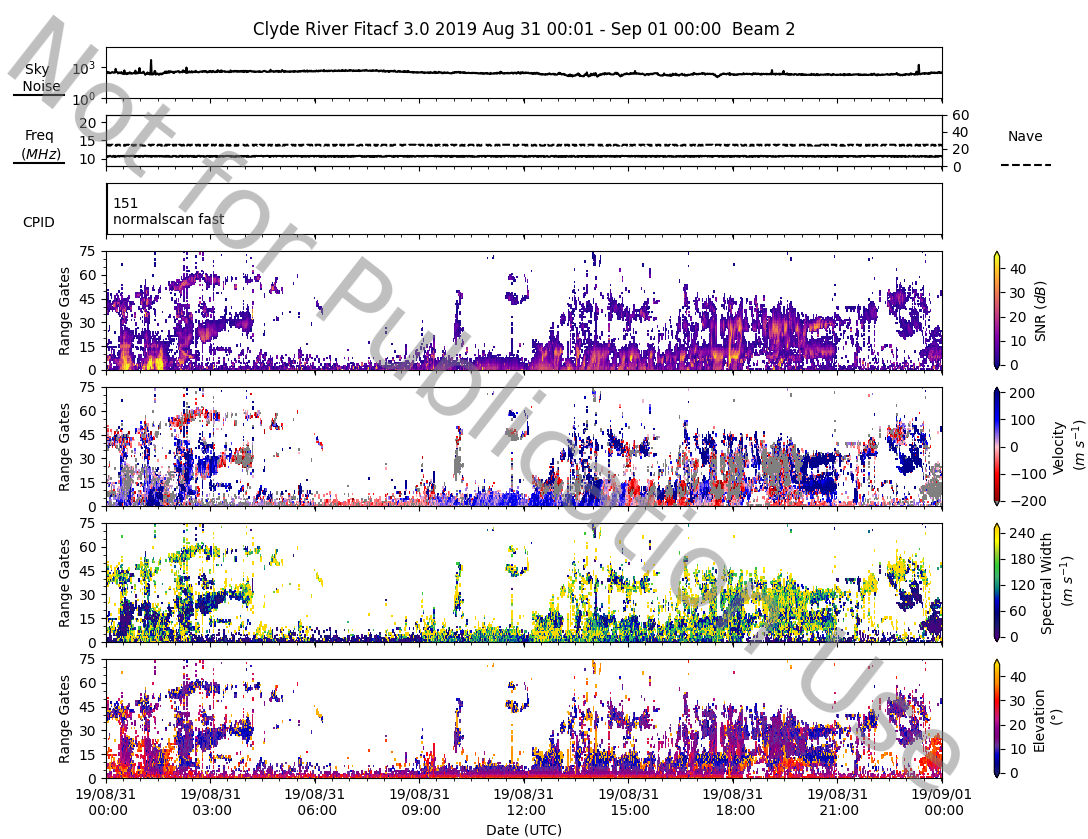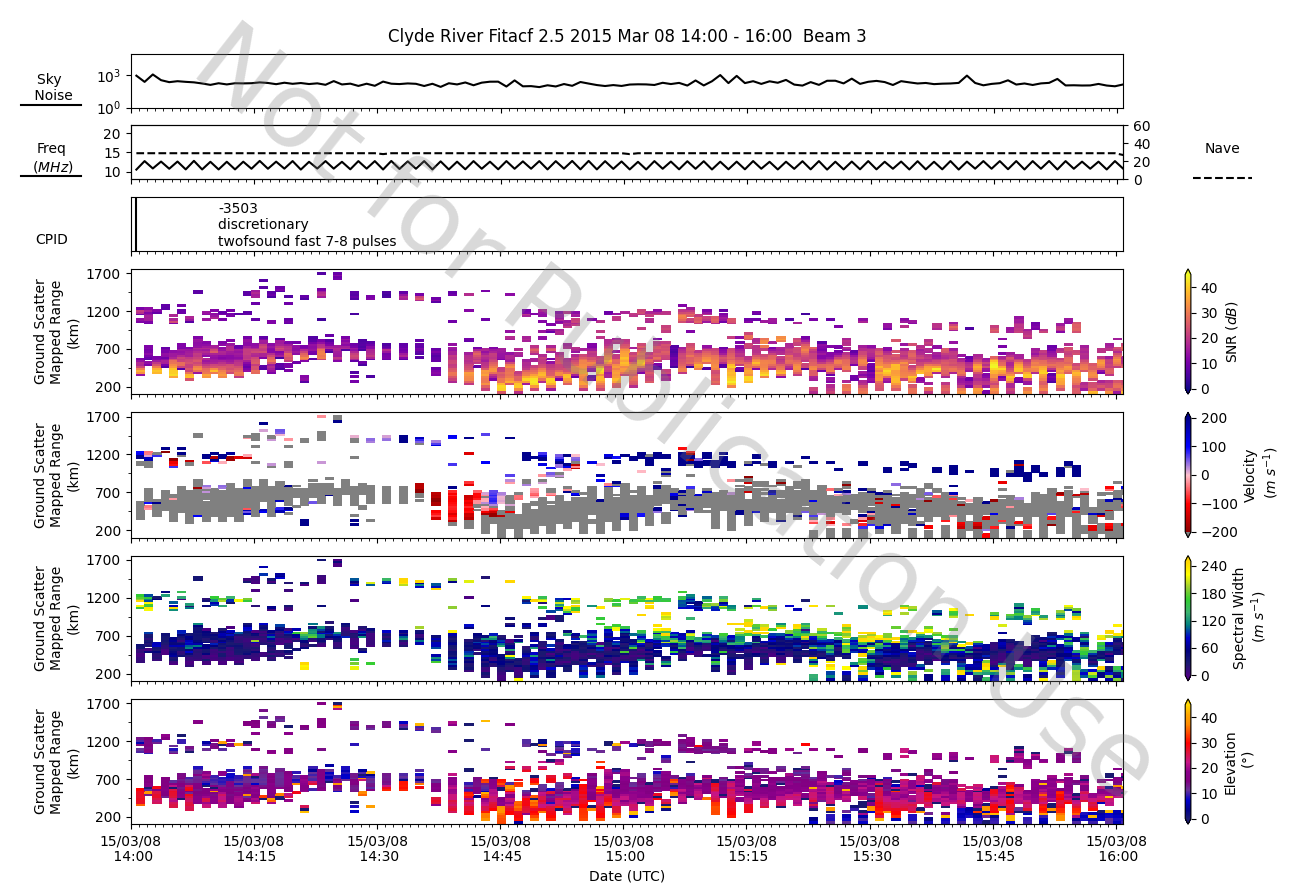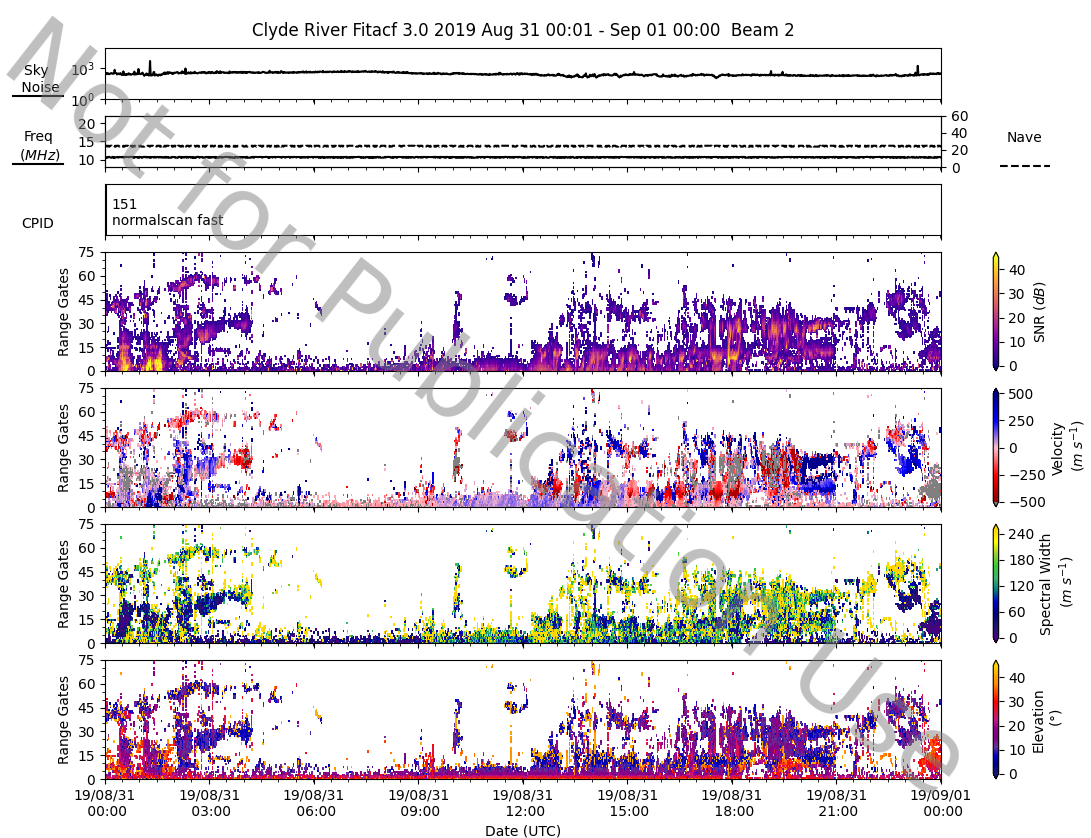# Summary plots

Summary plots in SuperDARN are a collection of set parameter plots from a FITACF file. The parameters typically in the plots are:

• Time-series plots:
• Sky Noise (`noise.sky`)
• Transmission Frequency and Number of averages (`tfreq` and `nav`)
• Control Program ID (`cp`)
• Range-time plots:
• Signal to Noise (`p_l`)
• Velocity (`v`)
• Spectral Width (`w_l`)
• Elevation (`elv`)

Note

Elevation (`elv`) is optional to plot and is set to be plotted, however, not all radars have elevation data. If the radar doesn't have elevation data then it is not plotted.

## Default Summary

With pyDARN and matplotlib imported, read in a FITACF with `pydarn.SuperDARNRead`, then call `plot_summary` with a chosen beam number. Here, we've loaded in some data from Clyde River and chosen beam 2:

``````import matplotlib.pyplot as plt

import pydarn

fitacf_file = "20190831.C0.cly.fitacf"

pydarn.RTP.plot_summary(fitacf_data, beam_num=2,
range_estimation=pydarn.RangeEstimation.RANGE_GATE)
plt.show()
``````

which gives:Note that ground scatter is displayed as default in the velocity panel. To disable it, you can use the option `groundscatter=false`.

In addition, we use `range_estimation=pydarn.RangeEstimation.RANGE_GATE` to set the y-axis to plot in range gates. Ground-Scatter Mapped Range is another type of axis you can use with range-time plots:

``````pydarn.RTP.plot_summary(fitacf_data, beam_num=3,
range_estimation=pydarn.RangeEstimation.GSMR)
``````In the example above, we can see the velocities are a little too high for the default colour scale. To change that, we need to create a python dictionary that redefines the boundaries:

``````boundaries={'v': [-500,500]}

pydarn.RTP.plot_summary(fitacf_data,beam_num=2,boundary=boundaries)
plt.show()

``````

which gives:You can change the boundaries on all of the panels by adding more keys to the dictionary. The keys must be named correctly to work, using the strings at the top of this tutorial.

Other common options include:

Parameter Action
channel=(int) Specify channel number (default=all)
watermark=(bool) True adds a 'not for publication' watermark
cmaps=(dict/str) Specifies the colour maps used in plotting
range_estimation=(RangeEstimation) Coordinates to use for the y-axis (See Coordinates)
For more options on how to modify plot_summary, take a look at the method in `rtp.py`.